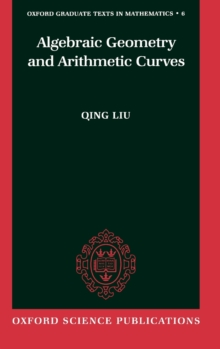Algebraic Geometry and Arithmetic Curves Hardback

Part of the Oxford Graduate Texts in Mathematics (0-19-961947-6) series

Description

This book is a general introduction to the theory of schemes, followed by applications to arithmetic surfaces and to the theory of reduction of algebraic curves.

The first part introduces basic objects such as schemes, morphisms, base change, local properties (normality, regularity, Zariski's Main Theorem).

This is followed by the more global aspect: coherent sheaves and a finiteness theorem for their cohomology groups.

Then follows a chapter on sheaves of differentials, dualizing sheaves, and Grothendieck's duality theory.

The first part ends with the theorem of Riemann-Roch and its application to the study of smooth projective curves over a field. Singular curves are treated through a detailed study of the Picard group. The second part starts with blowing-ups and desingularisation (embedded or not) of fibered surfaces over a Dedekind ring that leads on to intersection theory on arithmetic surfaces.

Castelnuovo's criterion is proved and also the existence of the minimal regular model.

This leads to the study of reduction of algebraic curves.

The case of elliptic curves is studied in detail. The book concludes with the funadmental theorem of stable reduction of Deligne-Mumford. The book is essentially self-contained, including the necessary material on commutative algebra.

The prerequisites are therefore few, and the book should suit a graduate student.

It contains many examples and nearly 600 exercises.

Information

• Format: Hardback
• Pages: 592 pages, numerous figures
• Publisher: Oxford University Press
• Publication Date:
• Category: Number theory
• ISBN: 9780198502845

£110.00

on all orders

Pick up orders

from local bookshops